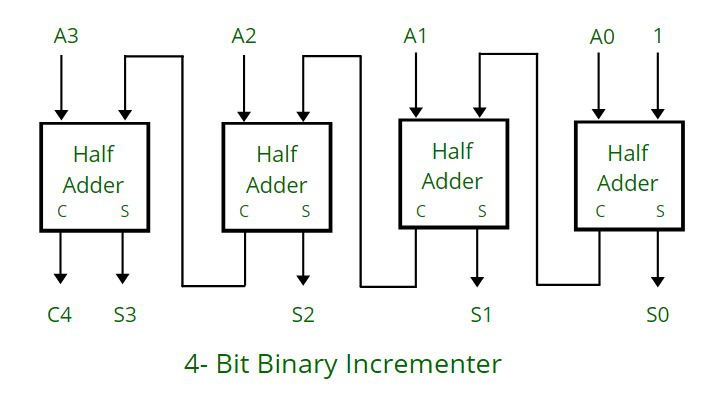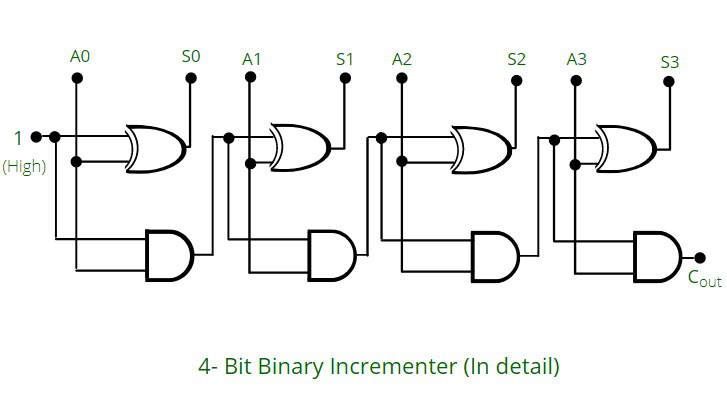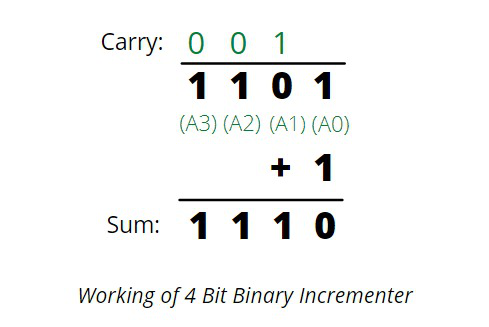Skip to content
Related Articles
4 Bit Binary Incrementer
• Last Updated : 29 Apr, 2021

What is 4 Bit Binary Incrementer ?
It adds 1 binary value to the existing binary value stored in the register or in other words we can simply say that it increases the value stored in the register by 1.
For any n-bit binary incrementer ,‘n’ refers to the storage capacity of the register which needs to be incremented by 1. So we require ‘n’ number of half adders . Thus, in case of 4 bit binary incrementer we require 4 half adders.Working:

• The half adders are connected one after the other , as it has 2 inputs and 2 outputs , so for the LSB ( least significant bit) half adder or the right most half adder is given 1 as direct input( first input) and A0 which is the first bit of the register (second input) , so we get the two output : sum (S0) and carry (C).
• The carry(C) from previous half adder is propagated to the next half adder, so the carry output of the previous half adder becomes the input of the next higher order half adder.
• So considering the case for 4 half adders the circuit gets in total 4 bits (A0, A1, A2, A3), 1 is added and we get an incremented output.Examples:

```(Refer to the circuit diagram from right to left for better understanding)

1. Input: 1010 ----> After using 4 bit binary incrementer ----> Output: 1011

1 0 1 0       (Comparing from the circuit 1 0 1 0 is A3, A2, A1, A0 respectively)
+ 1       (1 is added as seen in the diagram also, in the first half adder, 1 is taken as input)
_________
1 0 1 1       ( 1 0 1 1 , in the diagram are S3, S2, S1, S0 respectively)
_________```
```2. Input: 0010  ---> After using 4 bit binary incrementer ----> Output: 0011

0 0 1 0
+ 1
_________
0 0 1 1
_________```
```3. Input: 0011  ---> After using 4 bit binary incrementer ----> Output: 0100

0 0 1 1
+ 1
________
0 1 0 0
_________```

Attention reader! Don’t stop learning now. Get hold of all the important CS Theory concepts for SDE interviews with the CS Theory Course at a student-friendly price and become industry ready.

My Personal Notes arrow_drop_up